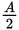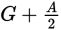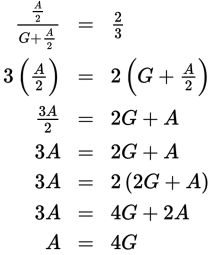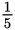# SAT Math Multiple Choice Question 838: Answer and Explanation

### Test Information

Question: 838

13. Amal, Geoffrey, and Julia each have some fireworks. If Amal gives half of his fireworksto Geoffrey, then Amal and Geoffrey will have fireworks in a 2:3 ratio. If Julia hasone-fifth as many fireworks as Geoffrey (after Amal gives half his fireworks to Geoffrey),then what is the ratio of the number of fireworks Amal has to the number of fireworksJulia has?

• A. 20:1
• B. 15:4
• C. 3:2
• D. There is insufficient information to determine the answer.

Explanation:

A

Difficulty: Hard

Category: Problem Solving and Data Analysis / Rates, Ratios, Proportions, and Percentages

Strategic Advice: When you see an answer choice indicating that there is insufficient information to answer the question, don't automatically assume that that choice is correct.

Getting to the Answer: Let A and G equal the number of fireworks that Amal and Geoffrey have. If Amal gives half of his fireworks to Geoffrey, then Amal will havefireworks left while Geoffrey will have. You're told that these two values will be in a 2:3 ratio, so set up a proportion and cross-multiply to simplify:While this doesn't give you the exact numbers for either Geoffrey or Amal, it does tell you that Amal has 4 times as many fireworks as Geoffrey. If Geoffrey, in turn, has 5 times as many fireworks as Julia (because the question states that Julia hasas many as Geoffrey), then Amal must have 4 × 5 = 20 times as many fireworks as Julia, which makes the ratio 20:1. Choice (A) is correct.